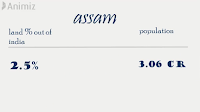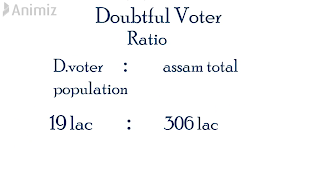# Financial Impact of NRC,CAB,CAA & NPR

So understanding the financial impact we have to dig the past data from Assam.

So now if we are talking about the areas & its population so we find that it consists 2.5% of total land in India & 3.06
crore population.& the expenses was done for the NRC was 1220 cr.
so now if we calculate the cost per person so we will get cost/person is rs 399(apporx).

so the projected total cost will be rs 54663 cr
that is huge...

But hay, its just beginning.lets see how much extra cost we need to cost we need to fulfill that .
From past data we found that 19 lac people were marked as doubtful voter in Assam.If we make a calculation so we find 1 person in every 16 person was a D.voter.Now as per the new regulation we were known to govt that only Muslims will be going to suffer that.But we are not sure about that at all, what is actually waiting for us as everyone is saying different kind of words. Ok for the calculation we take the minimum.Let assume only our Muslims brothers will be going to detention camp. So if we google it we can see
there are  201 million person in India having this community & which define 11% of total population of India.

So applying the ratio with that becomes 12562500 person.
Now govt need them to place them in detention camp.So if 1 detention camp cost 1 cr which can cover 100 people so govt need total 125625 detention camp which costs like 125625 cr .

Now the question is those 12562500 people definitely lost their jobs. suppose averagely they was earning minimum rs 2000/month cause this will include child,old person, not working women etc.If we calculate so we can see they were earning 30150 cr annually & this will be Nil & also govt need 30150 cr to feed them & nurture them.so it become 240588 cr or USD 3388 cr. weather till now we only discus about NRC cost. Now the question how much cost need to to conduct all CAA,NRC,NPR,CAB. If we take least so that will not be less than 2 times of projected NRC cost that is 240588*2 = 481176 cr. or USD 6776.
which represent 2.6% of our total GDP
6776/260000*100 =2.6%. & that is enough to collapse our  economy...
And this all money is going to spend from our tax.Do you want to know how much you to to paid to conduct this all thing
(4881176 cr/India total population)
=4881176 cr/137 cr
=rs 3512.
so finally you are going to spend this amount..

note: Given calculation can be less, can be very high depending on the situation. this post is not made to hurt any person, any community or any Act & law.
So now if we calculate all